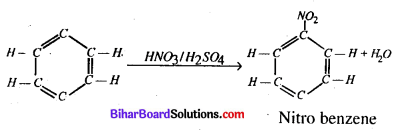# Bihar Board 12th Chemistry Model Question Paper 2 in English Medium

Bihar Board 12th Chemistry Model Papers

## Bihar Board 12th Chemistry Model Question Paper 2 in English Medium

Time : 3 Hours 15 Min
Full Marks: 70

Instructions for the candidates

1. Candidates are required to give answers in their own words as far as practicable.
2. Figures in the right hand margin indicates full marks.
3. While answering the questions, candidate should adhere to the words limit as far as practicable.
4. 15 Minutes of extra time has been allotted for the candidates to read the questions carefully.
5. This question paper is divided into two sections : Section -A and Section-B
6. In Section A, there are 35 objective type questions which are compulsory, each carrying 1 mark. Darken the circle with blue/black ball pen against the correct option on OMR Sheet provided to you. Do not use Whitener/Liquid/Blade/ Nail on OMR Sheet otherwise your result will be treated as invalid.
7. In section-B, there are 18 short answer type questions (each carrying 2 marks), out of which only 10 (ten) questions are to be answered Apart from this there are 06 Long Answer type questions (each carrying 5 marks), out of which 3 questions are to be answered.
8. Use of any electronic device is prohibited.

Objective Type Questions

In the following questions no. from 1 to 35, there is only one correct answer against each question. For each question, mark (darken) the correct answer on the OMR Sheet provided to you. (1 x 35 = 35)

Question 1.
Which among the following will show anisotropy ?
(a) Glass
(b) NaBr
(c) Plastic
(d) Rubber
(b) NaBr

Question 2.
Study the figure of a solid given below depicting the arrangement of particles. Which is the most appropriate term used for the figure ?(a) Isotropy
(b) Anisotropy
(c) Irregular
(d) Amorphous nature
(b) Anisotropy

Question 3.
Which of the following forms a molecular solid when solidified ?
(a) Calcium fluoride
(b) Silicon dioxide
(c) Carbon dioxide
(d) Sodium chloride
(c) Carbon dioxideQuestion 4.
Ionic solids conduct electricity in molten state but not in solid state because ……………..
(a) in molten state free ions are furnished which are not free to move
(b) in solid state in solid state ionic are hard, brittle and become soft in molten state
(c) all solids conduct electricity in molten state
(d) in solid state ions are converted to atoms which are insulators.
(a) in molten state free ions are furnished which are not free to move

Question 5.
The major binding force in diamond, silicon and quartz is ………………
(a) electrostatic force
(b) electrical attraction
(c) covalent bond force
(d) van der Waals force
(c) covalent bond force

Question 6.
Homogeneous mixture of two or more than two components is called …………….
(a) solute
(b) solvent
(c) both (a) and (b)
(d) solution
(d) solution

Question 7.
Which of the following is an example of gaseous solution ?
(a) Camphor is nitrogen gas
(b) Solution of hydrogen, in palladium
(c) Chloroform mixed with nitrogen gas .
(d) Both (a) and (c)
(d) Both (a) and (c)Question 8.
In a Daniell cell,
(a) the chemical energy liberated during the redox reaction is converted to electrical energy
(b) the electrical energy of the cell is converted to chemical energy
(c) the energy of the cell is utilised in conduction of the redox reaction
(d) the potential energy of the cell is converted into electrical energy.
(a) the chemical energy liberated during the redox reaction is converted to electrical energy

Question 9.
Which of the following is the correct cell representation for the given cell reaction ?
Zn H2SO4 → ZnSO4 + H2
(a) Zn|Zn2+||H+|H,
(b) Zn |Zn2+||H+, H,|Pt
(c) Zn |ZnSO4||H2SO4|Zn
(d) Zn|H2SO4||ZnSO4|H2
(b) Zn |Zn2+||H+, H,|Pt

Question 10.
When a chemical reaction takes place, during the course of the reaction the rate of reaction
(a) keeps on increasing with time
(b) remains constant with time
(c) keeps on decreasing with time
(d) shows irregular trend with time
(c) keeps on decreasing with timeQuestion 11.
For a reaction P + Q → 2R + S. Which of the following statements is incorrect ?
(a) Rate of disappearance of P = Rate of appearance of S
(b) Rate of disappearance of Q = 2 x Rate_of appearance of R
(c) Rate of disappearance of P = Rate of disapearance of Q
(d) Rate of disappearance of Q= -1/2 x Rate of appearance of R
(b) Rate of disappearance of Q = 2 x Rate_of appearance of R

Question 12.
After the reaction is over between adsorbed reactants, it is important to create space for the other reactant molecules to approach the surface and react. The process responsible for this is known as ………….
(a) sorption
(b) desorption
(c) physisorption
(d) chemisorption
(b) desorption

Question 13.
Powdered substances are more effective adsorbents than their crystalline form because
(a) adsorption is an exothermic process
(b) they become inert and do not react with the adsorbate
(c) the extent of adsorption increases with increase in surface area of the adsorbent
(c) the extent of adsorption increases with increase in surface area of the adsorbent

Question 14.
Which of the following example is not correctly matched ?
(a) Two most abudant elements-Fe, AI
(b) Two metals which occur in native state-Au, Pt
(c) Two metals which can occur in combined and native state both-Zn,Fe.
(d) None of these
(c) Two metals which can occur in combined and native state both-Zn,Fe.Question 15.
Which of the following is not the correct name of the formula of the ore given with it ?
(a) MgSO4.7H2O – Epsom salt
(b) CuCO3.Cu(OH)2 – Malachite
(c) KAlSi3Og– Feldspar
(d) MgCl2.6H2O – Dolomite
(d) MgCl2.6H2O – Dolomite

Question 16.
Nitrogen shows different oxidation states ranging from
(a) -3 to +5
(b) -5 to + 5
(c) 0 to -5
(d) -3 to +3
(a) -3 to +5

Question 17.
The oxidation state of nitrogen is highest in ……………….
(a) N3H
(b) NH3
(c)NH2OH
(d) N2H4
(a) N3HQuestion 18.
General electronic configuration of transition metals is ………………….
(a) (n-1)d1_10ns2
(b) ndwns2
(c) (n-1)d10ns2
(d) (n-1)d15ns2
(a) (n-1)d1_10ns2

Question 19.
Which one of the following is a ‘d-block element’ ?
(a)Gd
(b)Hs
(c) Es
(d) Cs
(b)Hs

Question 20.
Copper sulphate dissolves in ammonia due to the formation of
(a) Cu2O
(b) [Cu(NH3)4]SO4
(c) [Cu(NH3)4]OH
(d) [Cu(H2O)4]SO4
(b) [Cu(NH3)4]SO4

Question 21.
The numberofions given by [Pt(NH3)6]Cl4 inaqueous solution wil be ………………….
(a) two
(b) three
(c) five
(d) eleven
(c) five

Question 22.
Which of the following is a primary halide ?
(a) iso-Propyl iodide
(b) sec-Butyl iodide
(c) tert-Butyl bromide
(d) neo-Hexyl   chloride
(d) neo-Hexyl   chlorideQuestion 23.
Which of the following is not an allylic halide ?
(a) 4-Bromopent-2-ene
(b) 3-Bromo-2-methylbut-l-ene
(c) 1-Bromobut-2-ene
(d) 4-Bromobut-1-ene
(d) 4-Bromobut-1-ene

Question 24.
C5H12O is a monohydric alcohol. How many isomers of this alcohol are possible ? How many of these contain chiral centres as well as can exhibit enantiomerism ?
(a) 8 and 3
(b) 6 and 2
(c) 4 and 2
(d) 12 and 4
(a) 8 and 3

Question 25.
IUPAC name of(a) 3-propylbutan-1-o1
(b) 2-ethylpentan-1-o1
(c) 3-methyl hydroxyhexane
(d) 2-ethyl-2-propyl ethanol
(b) 2-ethylpentan-1-o1Question 26.
Which of the following structures is not correctly matched ?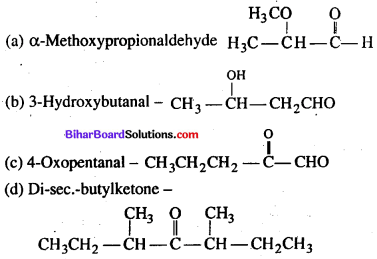(c)

Question 27.
Which of the following carbonyl compounds is most , polar ?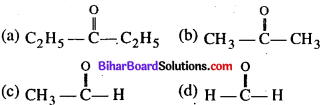(d)

Question 28.
Nitrogen atom of amino group is……
(a) sp
(b)sp2
(c) sp3
(d) sp3d
(c) sp3

Question 29.
C3H9N cannot represent
(a) 1° amine
(b) 2° amine
(c) 3° amine
(d) quaternary ammonium salt
(d) quaternary ammonium salt

Question 30.
The general formula of carbohydrates is……………….
(a) CnH2n+10
(b) CnH2nO
(c) Cn(H2O)n
(d)Cn(H2O)2n
(c) Cn(H2O)nQuestion 31.
Which of the following compounds is found abundantly in nature ?
(a) Fructose
(b) Starch
(c) Glucose
(d) Cellulose
(d) Cellulose

Question 32.
Glycogen, a naturally occuring polymert stored in animals is a
(a) Monosaccharide
(b) Disaccharide
(c) Trisaccharide
(d) Polysaccharide.
(d) Polysaccharide.

Question 33.
Which of the following is a homopolymer ?
(a) Bakelite
(b) Nylon 6, 6
(c) Neoprene
(d) Buna -S
(c) Neoprene

Question 34.
The use of chemicals for treatment of disease is called ………………….
(a) chemotherapy
(b) Physiotherapy
(c) angiotherapy
(d) polytherapy.
(a) chemotherapy

Question 35.
Which of the following is a criteria to classify drugs ?
(a) Chemical structure
(b) Molecular targets
(c) Drug action
(d) All of these.
(d) All of these.

Non-Objective Type Questions

In this Section, there are 18 Short answer type questions (each carrying 2 marks), out of which answer any 10 questions.  2 x 10 = 20

Question 1.
How will you distinguish between Lyophobic colloid and Lyophilic colloid ?
Lyophobic Colloids:

• They are irreversible in nature
• They are easily precipitated by addition of a small amount of suitable electrolyte.

Lyophilic Colloids :

• They are. reversible in nature.
• They are completely stable and do not easily precipitated.

Question 2.
Calculate the osmotic pressure of 5% solution of urea at 273 K.
If the pressure applied on the solution in greater than osmotic pressure then solvent starts passing from solution into solvent. This is called reverse osmosis. The Phenomenon of reverse osmosis is generally used for purification of sea water or hare water.Question 3.
Write IUPAC names of the following :
(a) K2[Ni(CN)4]
(b) [CoCl2(NH3)4]CI
K2 [Ni(CN)4] → Potassium Tetra Cyanonicilet (III)
CoCl2 (NH3)4 Cl → Tetramine Dichloride Cobalt (III) Chloride.

Question 4.
How would you convert
(a) aniline to bromobenzene
(b) ethanal to methanamine ?
(a) Aniline to Bromobenzene :(b) Ethanol to methanamine :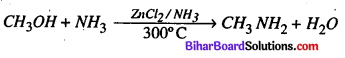Question 5.
Given reasons for the following :
(a) Formic acid is stronger than acetic acid.
(b) Aniline is less basic than methylamine.
(a) Formic acid is stronger than acetic acid – In every acid its acidity depends upon donation of proton from the solution .If the value of Ka is maximum, the acidity will be maxm-
HCOOH → Ka – 1.77 x 105
CH3COOH → Ka- 1.75 x 105
So, by the above equations it is clear that formic acid is more acidic than acetic acid.

(b) Aniline is less basic than methylamine -In aniline one pair of electron is present on nitrogen atom which is overlapped with n – electron in the ring. So, nitrogen atom is positively charged or there in lack of electrons due to this it is not in the state that it can give paired electrons to acid, in comparision with methylamine, due to this there in no overlaping. Thus, aniline is a weak base than methylamine.

Question 6.
Account for the following :
(a) The boiling point of ether is much lower than that of alcohol.
(b) Phenol is more acidic than alcohol.
(a) The boiling point of other is much lower than that of alcohol-In the case of alcohol hydrogen bond is present between the molecules, whereas in ether there is no hydrogen bond present between their molecules. nDue to this reason, the boiling point of ether is much lower than alcohol.

(b) Phenol is more acidic than alcohol-Phenol is generally a weak acid, but it has acidic property because it gives phenoscide ion in the solution.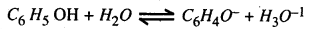In the above reaction the negative charge gives stability to the phenoscide ion.Question 7.
Write down the half cell reaction and cell reaction for the Daniel cell.
Zn(s) | Zn+2(aq) (1M) || Cu+2(aq) (1M) | Cu(s)
Zn (s) → Zn2+ (aq) + Ze (half cell reaction)
Cu++ (aq) + 2e → Cu (s) (half cell reaction)
———
Zn + Cu++ (1M) → Zn++ (1M) + Cu (Complete Cell reaction)

Question 8.
Account for the following .
(a) HI is stronger acid than HF
(b) The electron affinity of fluorine is less than that of chlorine.
(a) HI is stronger acid than HF – The bond length between HF is very small and since Fluorine is the most electronegative element of the periodic table Due to this reason HF is more electronegative which catches hydrogen strongly. On the other hand, bond-length between HI is very big because T is less electronegative than F. Due to this reason H+ ion comes out easily after Alteration.

(b) Since we know that the bond distance increases from I2 to I1 So the bond dissociation enthalpy should decrease, because of the increase in size of atom as we move from F to The F-F bond dissociation enthalpy is smaller than that of Cl-Cl and smaller than that of Br-Br. This is due to the reaseon than that of the F atom in very small and the there lone pairs of electrons on each f-atom repel the bond pair holding the F- atoms F2 molecule. I2< F2< Br2 < Cl2

Question 9.
Give definitions of the following with examples :
(a) Calcination
(b) Roasting
(a) Calcination → The process of heating a metal- rich arc to a high temperature to convert the metal into its oxide either in absence or insufficient supply of air is called calcination.
eq: Al2 O3 . 2H2O → Al2 O3 + 2H2O
2 Al (OH)3 → Al2 O3 + 3 H2O

(b) Roasting →The process of heating a finely ground arc to a high temperature in excess of air in called roasting.
Roasting converts the metal present in the ore to its oxide.
4FeS2 -1102 → 2Fe2d3 +8SO2
2Cu2S + 3O2 → 2Cu2O + 2SO2

Question 10.
Complete the following reactions :
(a) CHCl3 + ale. KOH + C6H5NH2
(b) CH3CH2OH + I2 + NaOH →
(a) CHCl3 + KOH+C6H5NH2 → C6H5NC+3KCl+3H20.
(b) CH3CH3OH + 412 + NaOH → CHI3 + NCOONa + 5Hl.Question 11.

• In this phenomenon, the concentration on the surface of adsorbent is different from that in the bulk.
• It occurs only at the surface of adsorpent.

Absortion :

• In this phenomenon, the concentration is same through out the material.
• It occurs throughtout the body of the material.

Question 12.
What is the difference between Schottky defect’ and ‘Frenkel defect’ ?
The difference between ‘Schottky defect’ and ‘Frenkel defect’ is stated below
Schottky defect :

• This type of defect is created when one positive ion and one negative ion are missing from their respective positions, leaving behind a pair of holes
• Maximum no. of Schottky defects in crystal minimizes the density
Ex-NaCl, kCl, CsC12KBrFrenkel defect:

•  This type defect is created when an ion leaves its correct lattice site & occupies an intersitial site.
• It does not give any charge in density. eg-Zns, AgCl, AgBr and Agl M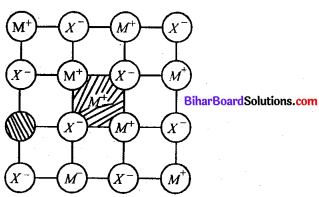Question 13.
When 10 gm of a non-volatile solute is dissolved in 100 gm of benzene, its boiling point is raised by 1°. What is the molecular mass of the solute ? (K4 for benzene – 2-53 Km-1)
Mass of solution (MB) = 100 g
Mass of solution (MA) = 100 g .
Mass of solution (NA) = 100 g
Raise in B.P. = ATb = 1°
Molecular mass of soluted (MB) = ?
Benzene (solution) Kh = 253 km-1 .
∵ ΔTb = Kh-m = 253 x $$\frac{10}{m_{B} \times 100} \times 1000$$
mB = 253gQuestion 14.
Define the following terms :
(a) Order of reaction
(b) Threshold energy.
Define the following terms
(a) Order of reaction – It is. an important parameter for every chemical reaction, which refers to the number of reacting particles.
Order of reaction – It is defined ass the sum of powers or exponents to which the concentration terms are raised in the rate law expression.
e.g. αA + bB → Products
Rate = K[A]m[B]m then, order of reaction is = (m + n)

(b) Threshold energy—The minimum amount of energy which the colliding particles must possess in order to bring about chemical reaction is called thresholod energy.

Question 15.
Define electrochemical equivalent.
Faraday’s 1st law of electrolysis – This law states that the mass of a substance produced at an electrode is directly proportional to the quantity of electricity passed.
m α Q  where,
m α I x t Q → quantity of elecricity.
m =Z x I x t
I → current in amperes.
t → time
Z → Electrochemical equivalent
Electrochemical – The amount of substance liberated at the electrode, when current of one ampere is passed through the electrolyte for one second.

Question 16.
If in a chemical reaction A+B product, rate law is given by R = K [A]1/2 [ B ]3/2, find the order of reaction.
A + B → production
$$\frac{d n}{d t}=R=K[A]^{1 / 2}[B]^{3 / 2}$$
So, order of reaction $$=\frac{1}{2}+\frac{3}{2}=\frac{4}{2}=2$$

Question 17.
What is Tyndall effect ? Discuss.
When a beam of light is passed through a colloidal solution, the beam is illuminated, such phenomena is not observed in true solution. Tyndall effect is observed when a beam of sunlight enter a earth room. Illuminating dust particles in beam scatter light.Question 18.
Give the name of two copper ores.

• CuFe S2
• Cu2 O

There are 06 long answer type questions (each carrying 05 marks), out of which answer any there questions.             (5 x 3 = 15)

Question 19.
(a) A first order reaction is 75% completed in 60 min. Find the half-life period of the reaction.
(b) Given one example each of zero order and first order reactions.
(a) We know that half life period $$t_{1 / 2}=\frac{0.693}{K}$$
Also, the first order expression is 2.303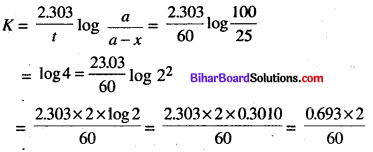Now, by putting the values of K in above equation.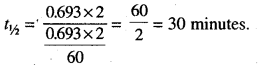(b) Examples of Zero-order reactionQuestion 20.
Describe the general characteristics of transition elements with special reference to the following :
(i) Formation of colour salt
(ii) Variable oxidation state.
(i) Formation of clour salt- Majority of transition metal compounds and cloured both in solid state as well as in aqeous solution. This is contrast to the compounds of respective (s and p-block) elements wich are usually colourless.

In a transition metal atom or oil, all the five d-orbits have the same energy i. e. these are degenerate orbitals. However three out of these (dxy, dyz, dzx) orbitals differ in shape from the other two (dx2y1dz2). When ligands both neutral or anionic approach these, species, the orbital no longer degenerate, and split into two sets of orbitals. This is called crytal field splitting one set with lower energy has three orbitals (dxy, d, dzx), where as the other set with slightly higher energy has two orbitals (dx2y2, dz2).

This, Promotion of one more electrons from a set of lower energy orbitals to a set of higher energy orbitals with in the same subshell is possible in case of transition elements with partly filled (n – 1) d-subshell. Since these two sets of d-orbital belonging to the same subshell. Have slightly different energy, the energy required to promote one or more electrons is small. Radiations of light corresponding to such a small amount of energy are available with it in the visible region of the spectrum.

Thus, when white light falls on such a transition element, some radiation corresponding to certain colour are absorbed and one are more electrons are raised from lower energy set of orbitals to higher energy set of orbitals. With the abosorption or radiations corresponding to specific colour from the white light a colour known as the complementary colour is observed or is transmitted. It may be noted the transmitted colour depends upon the colour which is observed for the electronic transition.(ii) The oxoanions of metals have co-valent mature in which oxygen is involved in the multiple bonding with the metal atom. This is responsible for the high oxidation state of the metal in oxoamine. For example-The elements Mn exibibits +7 oxidation state in permaganate ion (MnO4_)

Question 21.
Write IUPAC names of the following :
(i) CH3CONH2
(ii) (CH3)2CHCH2COCl
(iii) CH3 – O – C2H5
(iv) Ch3CH2CN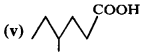(i) CH3 CONH2 → Ethanamide
(ii) (CH3)2 CHCH2 CoCl -3-Methyl butanoil Chloride.
(iii) CH3 – O – C2H5 – Methoxy ethane.
(iv) CH3CH2CN — Ethyl isocyanideQuestion 22.
What is polymerisation ? Define the terms addition polymerisation and condensation polymerisation. Give one example of each.
Polymerisation-Polymerization is a Process of reacting monomer molecules together in a chemical reaction to form polymer chains or three dimensional networks. Polymerisation occurs via a variety of reaction mechanisms that vary in colexity due to functional groups presnt in reacting compounds and their inherent steric effects.

There are following two types of polarization.

• Condensation Polymerization.
• Additional Polymerization-It is formed by the repeated addition of a large number of same or different monomers processing double and triple bonds.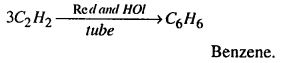(b) Condensation Polymerization- Condensation Polymers are formed by repeated condensation reaction between two bifunctional or trifunctional monomer units usually with the elimination of small molecules like water, alcohol, ammonia, Carbondioxide, hydrogen chloride, etc. and process by which condensation Polymers are formed is called condensation polymerization.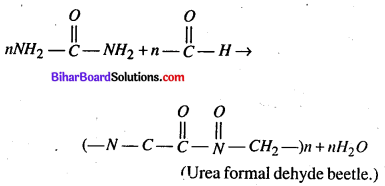Question 23.
A element A (atomic mass = 100) having 100 structure has unit cell edge length of 400 pm. Calculate the density of A and number of unit cells in 10 cm of A.
a (edge length) = 400 pm
Na = 6.02 x 1023
M = 100 gm / mole.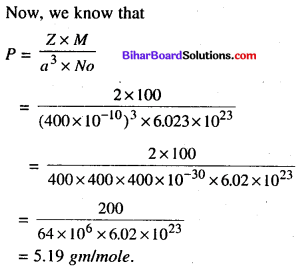Question 24.
Write the following giving chemical equations :
(i) Carbylamine reaction
(ii) Wurtz reaction.
(iii) Nitration of benzene